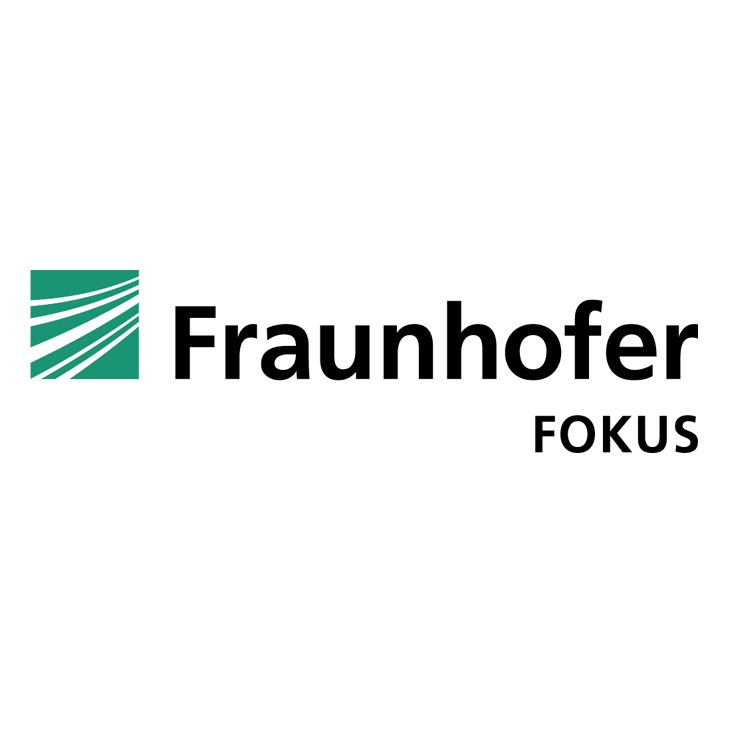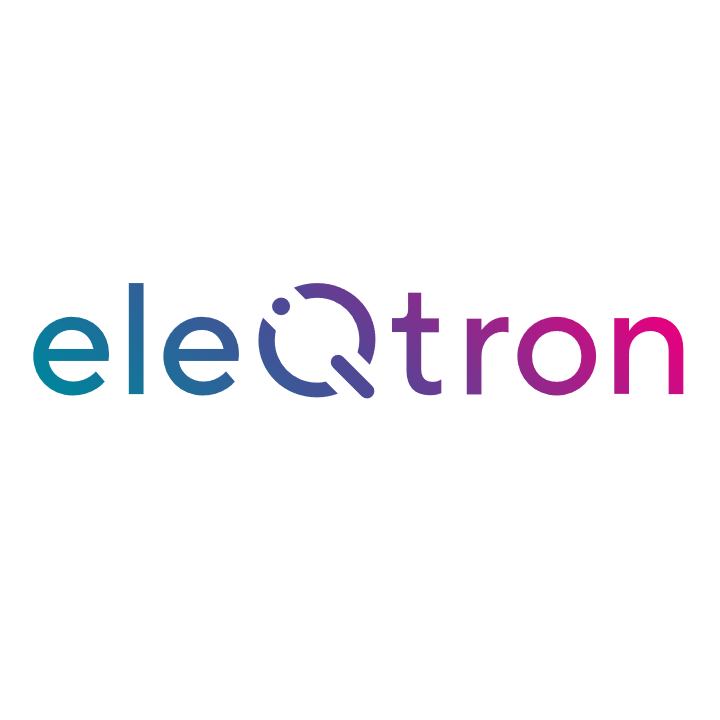## Qrisp

### The next generation of quantum algorithm development

Qrisp is a high-level programming language for creating and compiling quantum algorithms. Its structured programming model enables scalable development and maintenance.

Solve backtracking problems by leveraging quantum walksSynthesize arbitrarily complex diagonal hamiltoniansExplore parametrized algorithms with symbolic statevector simulation

\begin{align}\begin{aligned}\definecolor{qrispblue}{RGB}{32, 48, 111} \definecolor{textgray}{RGB}{68, 68, 68}\\\Large \textcolor{textgray}{ \begin{align} \frac{\ket{\texttt{hello}} + e^{i \textcolor{red}{\phi}} \ket{\texttt{world}}}{\sqrt{2}} \end{align} }\end{aligned}\end{align}

Solve the traveling salesman problem using quantum search# Key Features#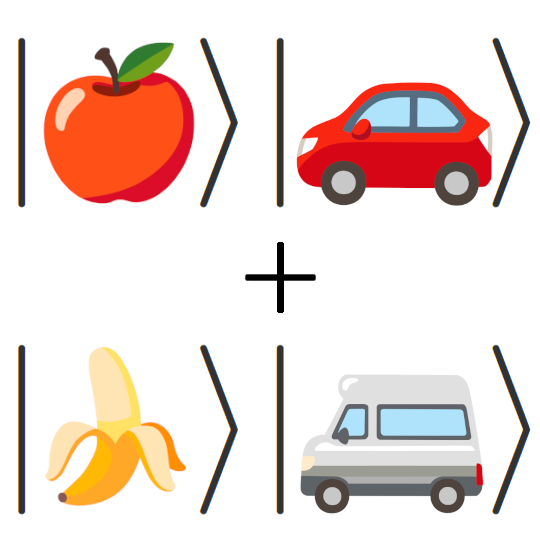Typed quantum variables
Qrisp algorithms are constituted of variables and functions instead of qubits and circuits, which helps you to structure your code and avoid technical debt.Automatic uncomputation
QuantumVariables can be uncomputed automatically once they are not needed anymore. The uncomputation module is tightly integrated with an advanced qubit resource managment system.Modularity
Automated qubit allocation allow separate modules to recycle qubit resources for eachother without intertwining the code. This feature facilitates interoperability of code written by respective domain experts.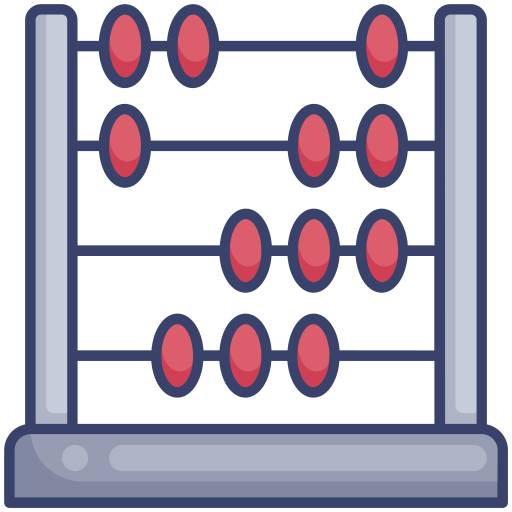Arithmetic
A smoothly integrated system of floating point arithmetic streamlines the development of non-trivial applications.Compatibility
Compilation results are circuit objects, implying they can be run on a variety of hardware providers such as IBM Quantum, Quantinuum, Rigetti etc. Further circuit processing is possible using circuit optimizers like PyZX.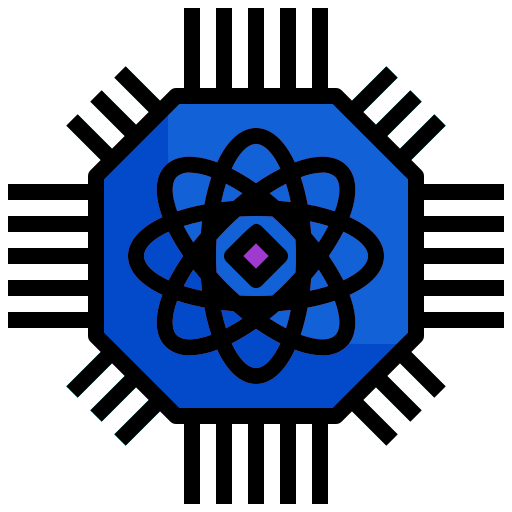Simulator
Qrisp ships with a high-performance simulator, that utilizes sparse matrices to store and process quantum states. This allows for the simulation of (some) quantum circuits involving 100+ qubits.

# Dive into Qrisp code#

Qrisp code can be significantly shorter and also more readable than the equivalent program in a gate based framework. Regardless, the compiled quantum circuits are in many cases more efficient since the compiler can leverage the code structure into assumptions about algorithmic structure, implying strong compilation optimizations. Below you find two code snippets that perform the same basic task: Multiplication of two n-bit integers. One is written using Qiskit and the other one utilizes Qrisp.from qiskit import (QuantumCircuit, QuantumRegister,
ClassicalRegister, Aer, execute)
from qiskit.circuit.library import RGQFTMultiplier
n = 6
a = QuantumRegister(n)
b = QuantumRegister(n)
res = QuantumRegister(2*n)
cl_res = ClassicalRegister(2*n)
qc = QuantumCircuit(a, b, res, cl_res)
for i in range(len(a)):
if 3 & 1<<i: qc.x(a[i])
for i in range(len(b)):
if 4 & 1<<i: qc.x(b[i])
qc.append(RGQFTMultiplier(n, 2*n),
list(a) + list(b) + list(res))
qc.measure(res, cl_res)
backend = Aer.get_backend('qasm_simulator')
counts_dic = execute(qc, backend).result().get_counts()
print({int(k, 2) : v for k, v in counts_dic.items()})
#Yields: {12: 1024}

from qrisp import QuantumFloat
n = 6
a = QuantumFloat(n)
b = QuantumFloat(n)
a[:] = 3
b[:] = 4
res = a*b
print(res)
#Yields: {12: 1.0}


Apart from simple scripts like the above, our tutorial showcases the utilization of Qrisp in solving the traveling salesman problem. This solution involves over 10 distinct QuantumVariables, with their respective qubits being repeatedly disentangled and repurposed for other variables. The presented approach scales better in the qubit count than the previously known QUBO based solution: $$\mathcal{O}(n \text{log}(n))$$ vs. $$\mathcal{O}(n^2)$$.

This example illustrates how Qrisp, as a high-level language, permits novel and scalable solutions to intricate problems and furthermore that high-level quantum programming languages will be an integral part of the future of quantum information science.

# Who is behind Qrisp#

Qrisp is an open-source project developed at Fraunhofer FOKUS, an industrial research facility based in Berlin. It is publicly funded by the German ministry of econmic affairs with the aim to enable commercial use of quantum computation. To achieve this, we aim to open this field of research to a broad audience of developers. Furthermore we are proud to announce that Qrisp will become a part of the Eclipse foundation!